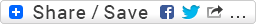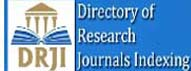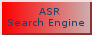• editor.aipublications@gmail.com
• Track Your Paper
• Contact Us
• ISSN: 2456-866X

# International Journal Of Chemistry, Mathematics And Physics(IJCMP)

### Indexing and Abstracting## Modelling of fouling in heat exchangers using the Artificial Neural Network Approach

Nadra Hussami , Hassan Al-Haj Ibrahim , Ahmad Safwat

Download | Downloads : 9 | Total View : 340

Journal : International Journal Of Chemistry, Mathematics And Physics(IJCMP)In this paper, modelling by neural networks was used for obtaining a model for the calculation of fouling factors in heat exchangers. The heat exchangers used in this study are a series of four exchangers where a model was obtained for each exchanger after due estimation of its heat load. The basic theme of this paper is the investigation of fouling factors and the determination of relevant indicators followed by combining design and operation factors along with fouling factors in a mathematical model that may be used for the calculation of the fouling factor. The devised model was tested for reliability and its accuracy in predicting new values for the fouling factor was greater than 98% in view of the design of the model Furthermore, the number of elements related to the design and operation was reduced to four developed formulae (developed factors) to which were added later the four factors selected as indicators of the occurrence of fouling. Both were then used as network input, whereas the output was the value of the fouling factor. The importance of this modelling lies in the fact that it enables the operator to continually predict the value of the fouling factor in heat exchangers and it assists him in taking appropriate measures to alleviate fouling effects ensuring thereby continuous operation of the unit and prevention of emergency shut downs.

naphtha hydro rating unit , heat exchanger ,fouling, moduling ,artificial neural network ANNW.